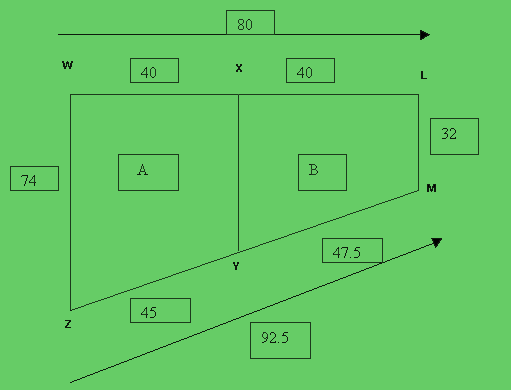Quandaries and Queries Pl. find the attached map.    1. Can u pl. find out the total square are of   WLMZ 2. Can u pl .find out the total square are of   WXYZ   --   { A } 3. Can u pl .find out the total square are of   XLMY   --    { B }   Pl .help me to find out the above.   Pl. suggest me the formula's.   When I tried using Pythagoras theorem, I got   XY as 38. Is it correct ?   But I am not getting Y perpendicular to WZ  correctly.   pl,. help me.   Thanks, Balachandar Hi Balachandar,There's not enough information -- we need to know if the lines WZ, XY, and ML are perpendicular to the line WL. If that is the case, Y would not be on the line ZM because parallel lines (WZ, XY, ML) would have to chop off equal segments ZY and YM. (Were the figure WZML a trapezoid then ZY = YM (which we could determine for you), and XY would have length (32 + 74)/2 = 53. Chris Go to Math Central